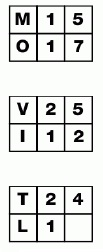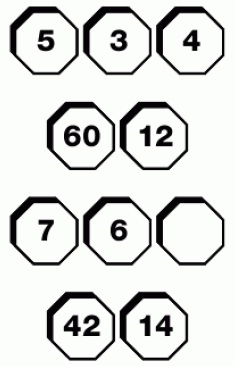##Puzzles Questions with Answers

1. What is missing from the empty square?Answer
 Ans = 6. Starting with each letter on the left add 2 to give the number in the next two boxes, for the next groups add 3 and 4.
2. Which number is missing?Answer
 Ans = 1. The product of the first row gives the first number in the second row. The sum of the numbers in the top row gives the second number. Repeat this for rows three and four.
12345678910

Page 1 of 10